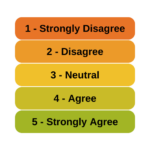# Ordinal Logistic Regression

### Member Training: Analyzing Likert Scale Data

August 31st, 2022 byIs it really ok to treat Likert items as continuous?And can you just decide to combine Likert items to make a scale? Likert-type data is extremely common—and so are questions like these about how to analyze it appropriately. (more…)

### Five Ways to Analyze Ordinal Variables (Some Better than Others)

April 14th, 2020 by

There are not a lot of statistical methods designed just to analyze ordinal variables.But that doesn’t mean that you’re stuck with few options.  There are more than you’d think.

Some are better than others, but it depends on the situation and research questions.

Here are five options when your dependent variable is ordinal.
(more…)

### How to Decide Between Multinomial and Ordinal Logistic Regression Models

March 11th, 2019 by

A great tool to have in your statistical tool belt is logistic regression.

It comes in many varieties and many of us are familiar with the variety for binary outcomes.

But multinomial and ordinal varieties of logistic regression are also incredibly useful and worth knowing.

They can be tricky to decide between in practice, however.  In some — but not all — situations you (more…)

### Opposite Results in Ordinal Logistic Regression, Part 2

July 22nd, 2013 by

I received the following email from a reader after sending out the last article: Opposite Results in Ordinal Logistic Regression—Solving a Statistical Mystery.

And I agreed I’d answer it here in case anyone else was confused.

Karen’s explanations always make the bulb light up in my brain, but not this time.

With either output,
The odds of 1 vs > 1 is exp[-2.635] = 0.07 ie unlikely to be  1, much more likely (14.3x) to be >1
The odds of £2 vs > 2 exp[-0.812] =0.44 ie somewhat unlikely to be £2, more likely (2.3x) to be >2

SAS – using the usual regression equation
If NAES increases by 1 these odds become (more…)

### Opposite Results in Ordinal Logistic Regression—Solving a Statistical Mystery

July 5th, 2013 by

A number of years ago when I was still working in the consulting office at Cornell, someone came in asking for help interpreting their ordinal logistic regression results.

The client was surprised because all the coefficients were backwards from what they expected, and they wanted to make sure they were interpreting them correctly.

It looked like the researcher had done everything correctly, but the results were definitely bizarre. They were using SPSS and the manual wasn’t clarifying anything for me, so I did the logical thing: I ran it in another software program. I wanted to make sure the problem was with interpretation, and not in some strange default or (more…)

### Logistic Regression Models for Multinomial and Ordinal Variables

January 14th, 2009 by

### Multinomial Logistic Regression

The multinomial (a.k.a. polytomous) logistic regression model is a simple extension of the binomial logistic regression model.  They are used when the dependent variable has more than two nominal (unordered) categories.

Dummy coding of independent variables is quite common.  In multinomial logistic regression the dependent variable is dummy coded into multiple 1/0 variables.  There is a variable for all categories but one, so if there are M categories, there will be M-1 dummy variables.  All but one category has its own dummy variable.  Each category’s dummy variable has a value of 1 for its category and a 0 for all others.  One category, the reference category, doesn’t need its own dummy variable as it is uniquely identified by all the other variables being 0.

The multinomial logistic regression then estimates a separate binary logistic regression model for each of those dummy variables.  The result is (more…)